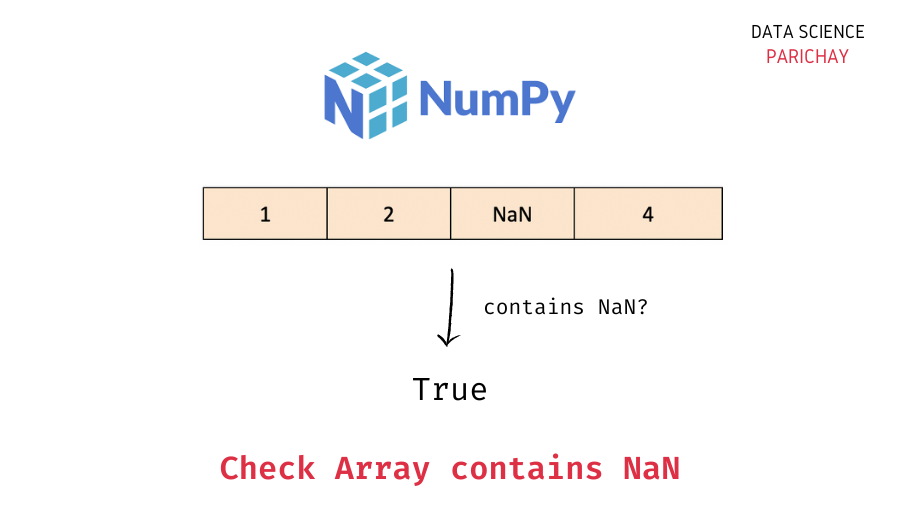# Numpy – Check If an Array contains a NaN value

When working with Numpy arrays, it can happen that they contain one or more NaN (not a number) values. In this tutorial, we will look at how to check if an array contains a NaN value or not.

## Steps to check if a Numpy array contains a NaN value

To check if an array contains a NaN value or not, use a combination of the `numpy.isnan()` function and the Python built-in `any()` function. The idea is to essentially check whether any value in the array is NaN or not.

Use the following steps –

1. Apply the `numpy.isnan()` function to the entire array, this will result in a boolean array with `True` for the values that are NaN and `False` for the values that are not NaN.
2. Then, apply the `any()` function on the above boolean array to check if there are any `True` values in the above array.

The following is the syntax –

```import numpy as np
# check if array contains a NaN value
any(np.isnan(ar))```

Let’s now look at some examples of using the above syntax –

### Example 1 – Check if a Numpy array has any NaN Values using `numpy.isnan()`

Let’s create a Numpy array containing a NaN value and use the above method to see if it gives us the correct result or not.

```import numpy as np

# create an array
ar = np.array([1, 2, 3, np.nan, 5])
# result of np.isnan
print(np.isnan(ar))
# check if the array contains any nan values
print(any(np.isnan(ar)))```

Output:

```[False False False  True False]
True```

In the above example, we print the result of the `np.isnan()` function, which you can see is a boolean array. We then use the `any()` function to check if there are any `True` values in the boolean array.

📚 Data Science Programs By Skill Level

Introductory

Intermediate ⭐⭐⭐

🔎 Find Data Science Programs 👨‍💻 111,889 already enrolled

Disclaimer: Data Science Parichay is reader supported. When you purchase a course through a link on this site, we may earn a small commission at no additional cost to you. Earned commissions help support this website and its team of writers.

We get `True` as the output which indicates that there is at least one NaN value present in the array.

### Example 2 – Numpy array without any NaN values

Let’s now create a Numpy array without any NaN values and apply the above method to see if we get the correct result or not.

```import numpy as np

# create an array
ar = np.array([1, 2, 3, 4, 5])
# result of np.isnan
print(np.isnan(ar))
# check if the array contains any nan values
print(any(np.isnan(ar)))```

Output:

```[False False False False False]
False```

We get `False` as the output of the `any()` function. This means that are were no `True` values in the array resulting from `np.isnan()` which you can verify from the printed array.

If, on the other hand, you want to check whether all the values in a numpy array are NaN or not, use the Python built-in `all()` function instead of the `any()` function.

```import numpy as np

# create an array
ar = np.array([np.nan, np.nan, np.nan])
# result of np.isnan
print(np.isnan(ar))
# check if the array contains only nan values
print(all(np.isnan(ar)))```

Output:

```[ True  True  True]
True```

We get `True` as the output.

For more on the numpy `isnan()` function, refer to its documentation.

You might also be interested in –

••